# CBSE Class 12 Physics Chapter 6 Revision Notes

Chapter 6: Electromagnetic Induction Revision Notes

• Magnetic Flux: The total number of magnetic lines of force passing normally through any surface equals the magnetic flux associated with it. It’s a number with a scalar value.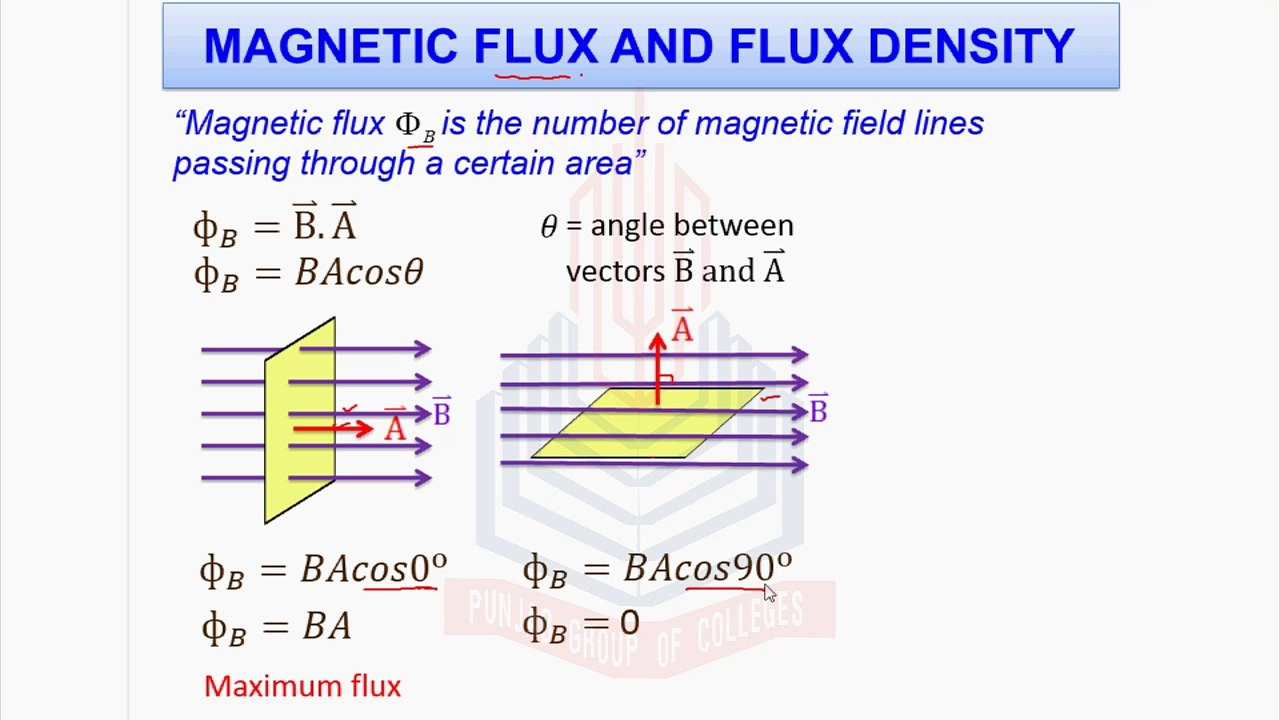Source

• Electromagnetic Induction (EMI) is the phenomenon of generating current or emf by changing the magnetic flux.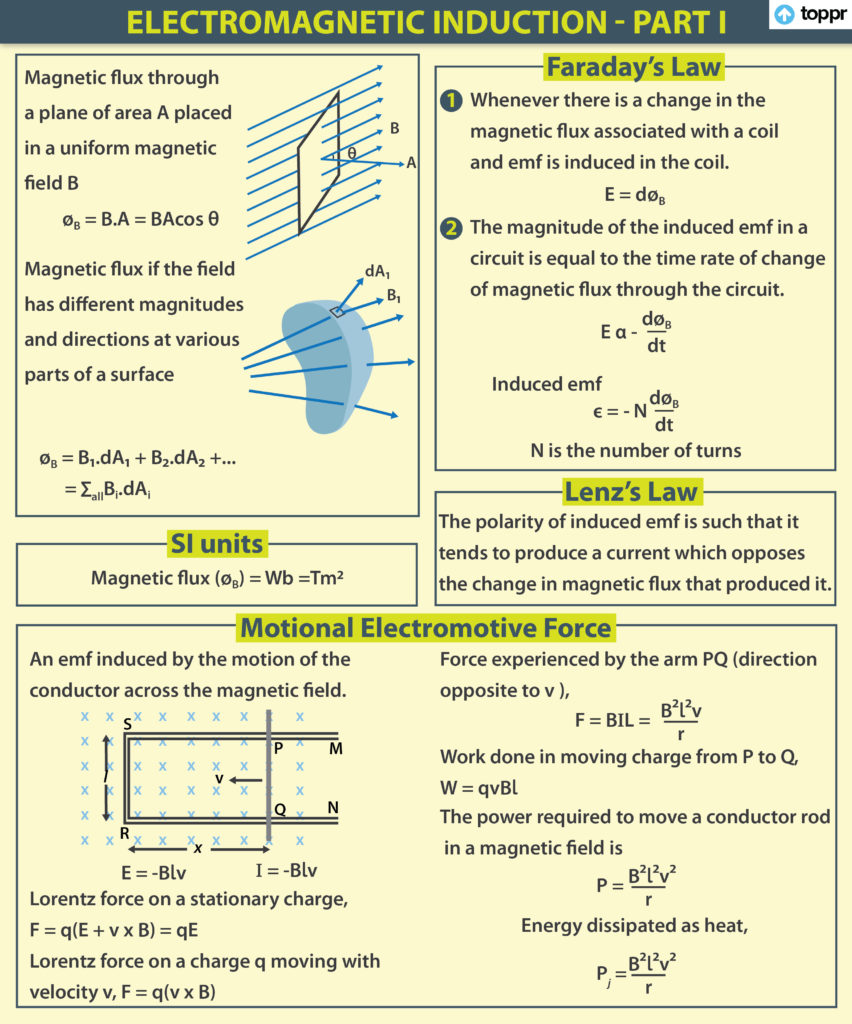Source

## Electromagnetic Induction and Faraday’s Law

• The First Law:

When the magnetic flux in a closed loop or circuit changes, an emf is generated in the loop or circuit that lasts as long as the flux is changing.

• The Second Law:

The rate of change of magnetic flux in a closed loop or circuit is directly proportional to the induced emf in the closed loop or circuit.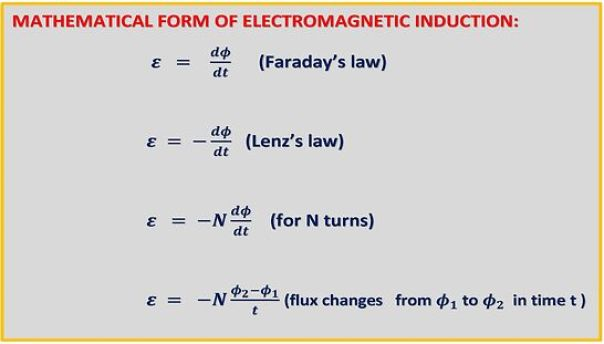Source

• Motional Emf describes the potential difference induced in a conductor of length l moving with velocity v in a direction perpendicular to magnetic field B.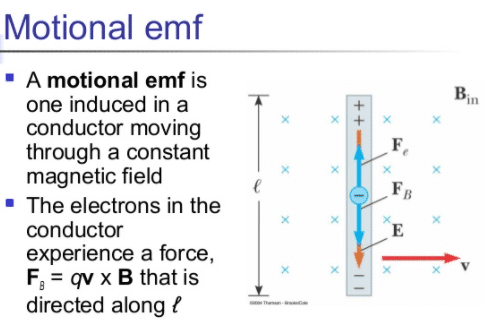Source

• The Right Hand Rule of Fleming:

If the right hand’s thumb, forefinger, and middle finger are stretched perpendicular to each other, the forefinger points in the direction of the magnetic field, the thumb points in the direction of magnetic force, and the middle finger points in the direction of the induced current in the conductor.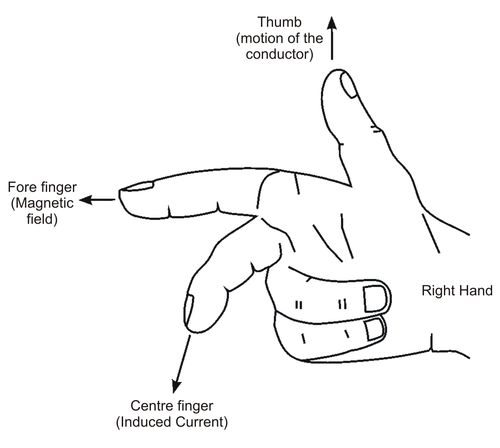Source

]]>